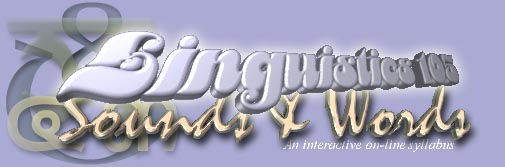## Consonantal Natural Classes

See if you can recognize the natural classes to which the following consonantal sounds belong.

1. To what natural class do the following consonants belong?
2.  p b t d k g

3. To what natural class do the following consonants belong?
4.  p b m f v b

5. To what natural class do the following consonants belong?
6.  s z Ś — x ď

7. To what natural class do the following consonants belong?
8.  m n ň ü

9. To what natural class do the following consonants belong?
10.  m n ň r l j w ü

11. To what natural class do the following consonants belong?
12.  j w

13. To what natural class do the following consonants belong?
14.  r l

15. To what natural class do the following consonants belong?
16.  m b v ö d z g ď — l r

17. To what natural class do the following consonants belong?
18.  p f Ę s t Ś “ k h

19. To what natural class do the following consonants belong?
20.  t d s z n

21. To what natural class do the following consonants belong?
22.  Ś — ň j

23. To what natural class do the following consonants belong?
24.  s Ś “ — z ”

25. To what natural class do the following consonants belong?
26.  k g x ď ü

27. To what natural class do the following consonants belong?
28.  pß bß tß dß kß gß

29. To what natural class do the following consonants belong?
30.  p' b' t' d' f' v'

31. To what natural class do the following consonants belong?
32.  “ ”

| Linguistics 110 | Linguistics Program | Linguistics 105 | Next > |